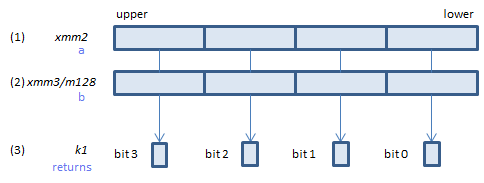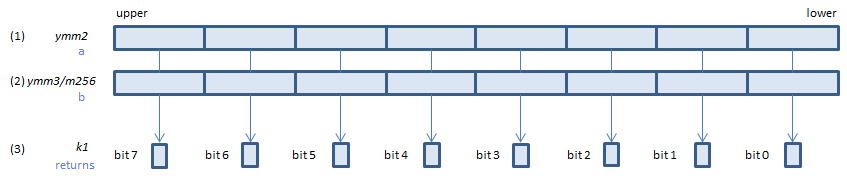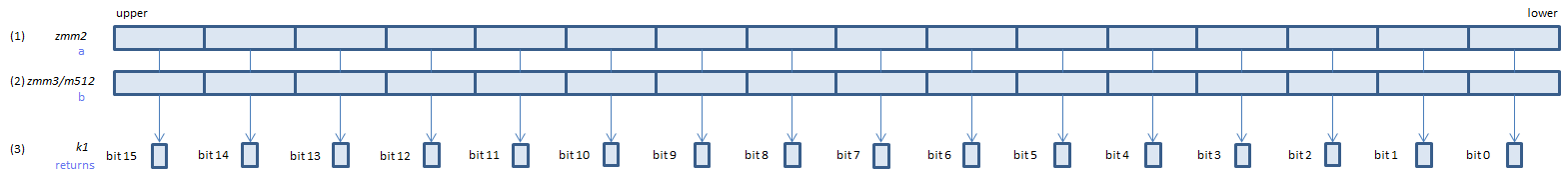﻿ vptestnmd

## VPTESTNMD - Packed TEST Nand Mask Dword

VPTESTNMD k1{k2}, xmm2, xmm3/m128/m32bcst    (V5+VLFor each DWORD calculate bitwise (1) & (2), if all the bits of the result are 0, set 1, else set 0, to the corresponding bit of (3).
If k2 bit is 0, the calculation is not done and the corresponding bit of (3) is set to zero. Upper bits of (3) are zero cleared.
VPTESTNMD k1{k2}, ymm2, ymm3/m256/m32bcst    (V5+VL